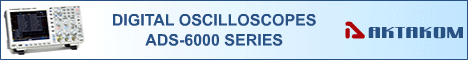Search

# HP-9060 Oscilloscope Probe 60MHz Selectable X1/Ref/X10

 The HP-9060 is a passive high impedance oscilloscope probe designed and calibrated for use with instruments having an input impedance of 1MΩ. Switch Selectable x1/ x10/ Ref Probe.

The HP-9060 is a passive high impedance oscilloscope probe designed and calibrated for use with instruments having an input impedance of 1MΩ. Switch Selectable x1/ x10/ Ref Probe.

X1 position:

• Input Impedance: R= 1MOhm, C= 68pf
• Bandwidth= 6MHz
• Max Voltage In(Vin)= 600V

X10 position:

• Input Impedance: R=10MOhm, C=18pf
• Bandwidth= 60MHz
• Max Voltage In(Vin)=600V

Back to the section